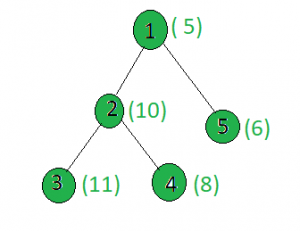# Find the node whose sum with X has maximum set bits

• Last Updated : 20 Apr, 2021

Given a tree, and the weights of all the nodes and an integer x, the task is to find a node i such that weight[i] + x has the maximum set bits. If two or more nodes have the same count of set bits when added with x then find the one with the minimum value.
Examples:

Input:x = 15
Output:
Node 1: setbits(5 + 15) = 2
Node 2: setbits(10 + 15) = 3
Node 3: setbits(11 + 15) = 3
Node 4: setbits(8 + 15) = 4
Node 5: setbits(6 + 15) = 3

Approach: Perform dfs on the tree and keep track of the node whose sum with x has maximum set bits. If two or more nodes have equal count of set bits then choose the one with the minimum number.
Below is the implementation of the above approach:

## C++

 `// C++ implementation of the approach``#include ``using` `namespace` `std;` `int` `maximum = INT_MIN, x, ans = INT_MAX;` `vector<``int``> graph;``vector<``int``> weight(100);` `// Function to perform dfs to find``// the maximum set bits value``void` `dfs(``int` `node, ``int` `parent)``{``    ``// If current set bits value is greater than``    ``// the current maximum``    ``int` `a = __builtin_popcount(weight[node] + x);``    ``if` `(maximum < a) {``        ``maximum = a;``        ``ans = node;``    ``}` `    ``// If count is equal to the maximum``    ``// then choose the node with minimum value``    ``else` `if` `(maximum == a)``        ``ans = min(ans, node);` `    ``for` `(``int` `to : graph[node]) {``        ``if` `(to == parent)``            ``continue``;``        ``dfs(to, node);``    ``}``}` `// Driver code``int` `main()``{``    ``x = 15;` `    ``// Weights of the node``    ``weight = 5;``    ``weight = 10;``    ``weight = 11;``    ``weight = 8;``    ``weight = 6;` `    ``// Edges of the tree``    ``graph.push_back(2);``    ``graph.push_back(3);``    ``graph.push_back(4);``    ``graph.push_back(5);` `    ``dfs(1, 1);` `    ``cout << ans;` `    ``return` `0;``}`

## Java

 `// Java implementation of the approach``import` `java.util.*;` `class` `GFG``{` `static` `int` `maximum = Integer.MIN_VALUE, x, ans = Integer.MAX_VALUE;` `static` `Vector> graph = ``new` `Vector>();``static` `Vector weight = ``new` `Vector();` `//number of set bits``static` `int` `__builtin_popcount(``int` `x)``{``    ``int` `c = ``0``;``    ``for``(``int` `i = ``0``; i < ``60``; i++)``    ``if``(((x>>i)&``1``) != ``0``)c++;``    ` `    ``return` `c;``}` `// Function to perform dfs to find``// the maximum value``static` `void` `dfs(``int` `node, ``int` `parent)``{``    ``// If current set bits value is greater than``    ``// the current maximum``    ``int` `a = __builtin_popcount(weight.get(node) + x);``    ``if` `(maximum < a)``    ``{``        ``maximum = a;``        ``ans = node;``    ``}` `    ``// If count is equal to the maximum``    ``// then choose the node with minimum value``    ``else` `if` `(maximum == a)``        ``ans = Math.min(ans, node);``        ` `    ``for` `(``int` `i = ``0``; i < graph.get(node).size(); i++)``    ``{``        ``if` `(graph.get(node).get(i) == parent)``            ``continue``;``        ``dfs(graph.get(node).get(i), node);``    ``}``}` `// Driver code``public` `static` `void` `main(String args[])``{``    ``x = ``15``;` `    ``// Weights of the node``    ``weight.add(``0``);``    ``weight.add(``5``);``    ``weight.add(``10``);;``    ``weight.add(``11``);;``    ``weight.add(``8``);``    ``weight.add(``6``);``    ` `    ``for``(``int` `i = ``0``; i < ``100``; i++)``    ``graph.add(``new` `Vector());` `    ``// Edges of the tree``    ``graph.get(``1``).add(``2``);``    ``graph.get(``2``).add(``3``);``    ``graph.get(``2``).add(``4``);``    ``graph.get(``1``).add(``5``);` `    ``dfs(``1``, ``1``);` `    ``System.out.println( ans);``}``}` `// This code is contributed by Arnab Kundu`

## Python3

 `# Python implementation of the approach``from` `sys ``import` `maxsize` `maximum, x, ans ``=` `-``maxsize, ``None``, maxsize` `graph ``=` `[[] ``for` `i ``in` `range``(``100``)]``weight ``=` `[``0``] ``*` `100` `# Function to perform dfs to find``# the maximum set bits value``def` `dfs(node, parent):``    ``global` `x, ans, graph, weight, maximum` `    ``# If current set bits value is greater than``    ``# the current maximum``    ``a ``=` `bin``(weight[node] ``+` `x).count(``'1'``)` `    ``if` `maximum < a:``        ``maximum ``=` `a``        ``ans ``=` `node` `    ``# If count is equal to the maximum``    ``# then choose the node with minimum value``    ``elif` `maximum ``=``=` `a:``        ``ans ``=` `min``(ans, node)` `    ``for` `to ``in` `graph[node]:``        ``if` `to ``=``=` `parent:``            ``continue``        ``dfs(to, node)` `# Driver Code``if` `__name__ ``=``=` `"__main__"``:` `    ``x ``=` `15` `    ``# Weights of the node``    ``weight[``1``] ``=` `5``    ``weight[``2``] ``=` `10``    ``weight[``3``] ``=` `11``    ``weight[``4``] ``=` `8``    ``weight[``5``] ``=` `6` `    ``# Edges of the tree``    ``graph[``1``].append(``2``)``    ``graph[``2``].append(``3``)``    ``graph[``2``].append(``4``)``    ``graph[``1``].append(``5``)` `    ``dfs(``1``, ``1``)` `    ``print``(ans)` `# This code is contributed by``# sanjeev2552`

## C#

 `// C# implementation of the approach``using` `System;``using` `System.Collections.Generic;` `class` `GFG``{` `static` `int` `maximum = ``int``.MinValue, x,ans = ``int``.MaxValue;` `static` `List> graph = ``new` `List>();``static` `List<``int``> weight = ``new` `List<``int``>();` `// number of set bits``static` `int` `__builtin_popcount(``int` `x)``{``    ``int` `c = 0;``    ``for``(``int` `i = 0; i < 60; i++)``    ``if``(((x>>i)&1) != 0)c++;``    ` `    ``return` `c;``}` `// Function to perform dfs to find``// the maximum value``static` `void` `dfs(``int` `node, ``int` `parent)``{``    ``// If current set bits value is greater than``    ``// the current maximum``    ``int` `a = __builtin_popcount(weight[node] + x);``    ``if` `(maximum < a)``    ``{``        ``maximum = a;``        ``ans = node;``    ``}` `    ``// If count is equal to the maximum``    ``// then choose the node with minimum value``    ``else` `if` `(maximum == a)``        ``ans = Math.Min(ans, node);``        ` `    ``for` `(``int` `i = 0; i < graph[node].Count; i++)``    ``{``        ``if` `(graph[node][i] == parent)``            ``continue``;``        ``dfs(graph[node][i], node);``    ``}``}` `// Driver code``public` `static` `void` `Main()``{``    ``x = 15;` `    ``// Weights of the node``    ``weight.Add(0);``    ``weight.Add(5);``    ``weight.Add(10);``    ``weight.Add(11);;``    ``weight.Add(8);``    ``weight.Add(6);``    ` `    ``for``(``int` `i = 0; i < 100; i++)``    ``graph.Add(``new` `List<``int``>());` `    ``// Edges of the tree``    ``graph.Add(2);``    ``graph.Add(3);``    ``graph.Add(4);``    ``graph.Add(5);` `    ``dfs(1, 1);` `    ``Console.Write( ans);``}``}` `// This code is contributed by mits`

## Javascript

 ``
Output:
`4`

Complexity Analysis:

• Time Complexity : O(N).
In dfs, every node of the tree is processed once and hence the complexity due to the dfs is O(N) if there are total N nodes in the tree. Also, for processing each node the builtin_popcount() function is used which has a complexity of O(c) where c is a constant and since this complexity is constant, it does not affect the overall time complexity. Therefore, the time complexity is O(N).
• Auxiliary Space : O(1).
Any extra space is not required, so the space complexity is constant.

My Personal Notes arrow_drop_up